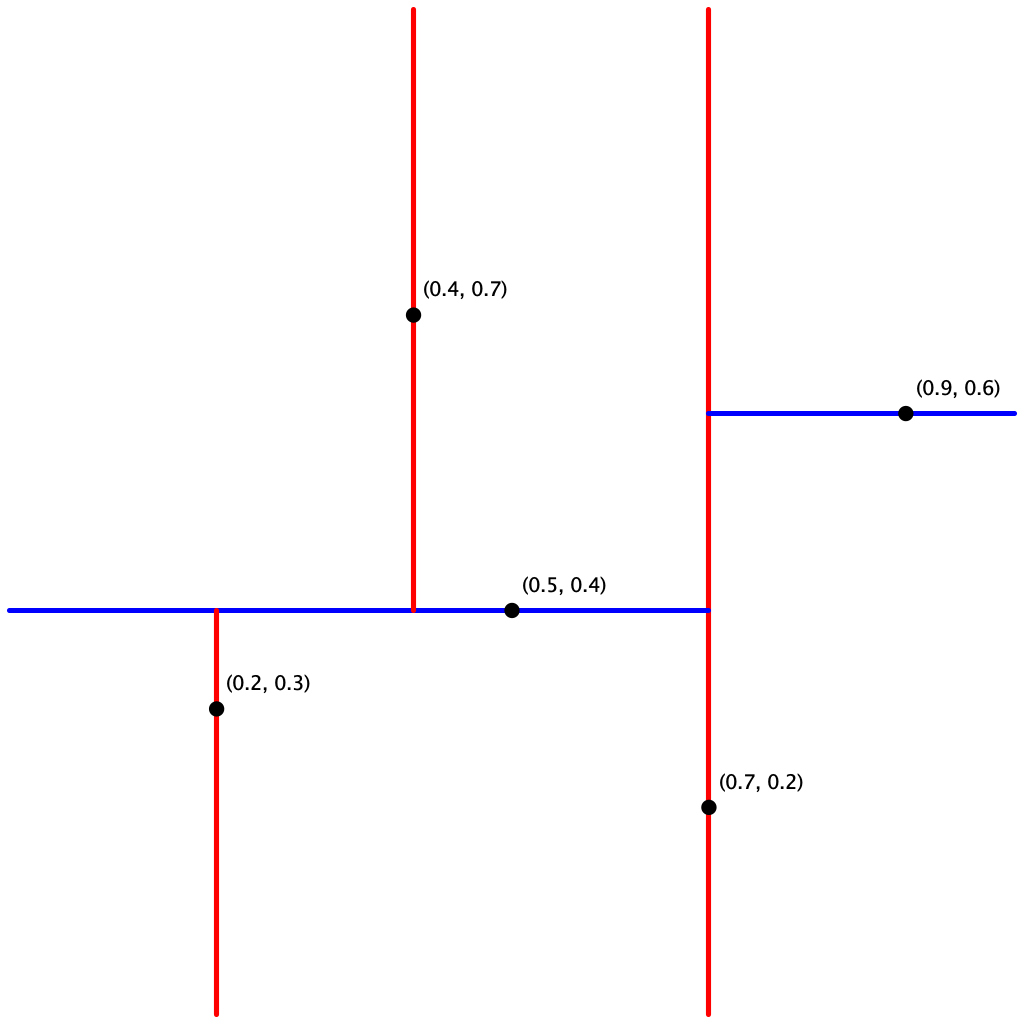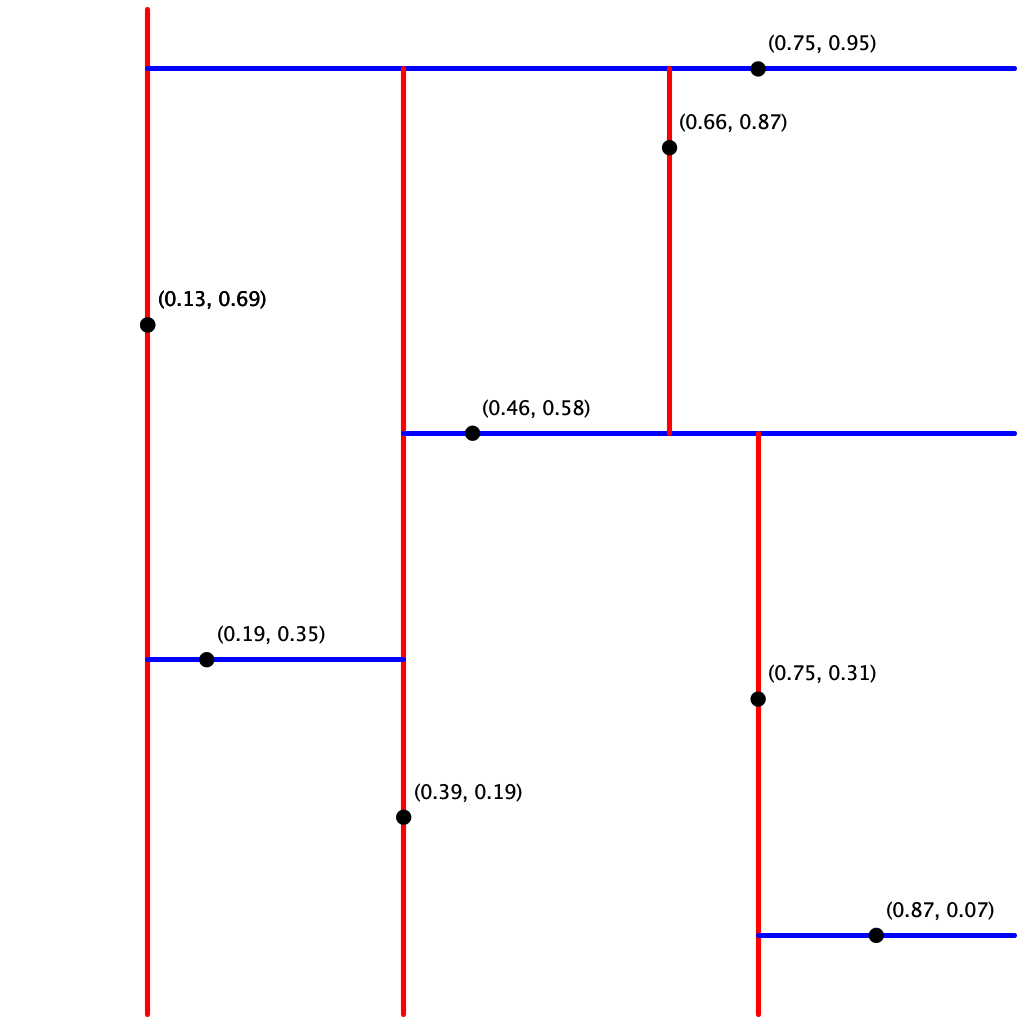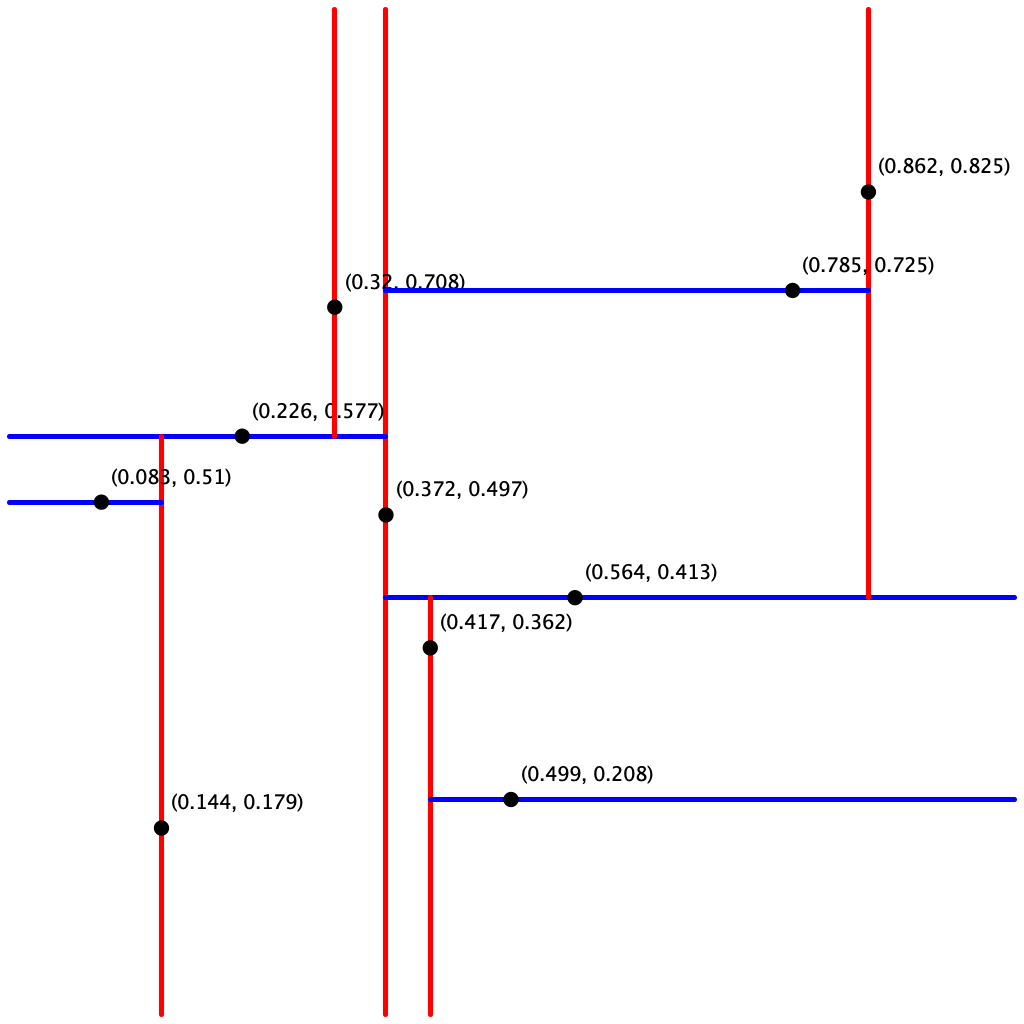Is a point on the boundary of a rectangle considered inside it? Do two rectangle intersect if they have just one point in common? Yes and yes, consistent with the implementation of RectHV.java.

Can I use the distanceTo() method in Point2D and RectHV? No, you may use only the subset of the methods listed. You should be able to accomplish the same result (more efficiently) with distanceSquaredTo().

Can I use the X_ORDER() and Y_ORDER() comparators in Point2D? No, you may use only the subset of the methods listed. You should be able to accomplish the same result by calling the methods x() and y().

What should I do if a point is inserted twice in the data structure? The data structure represents a symbol table, so you should replace the old value with the new value.

What should points() return if there are no points in the data structure? What should range() return if there are no points in the range? The API says to return an Iterable<Point2D>, so you should return an iterable with zero points.

What should nearest() return if there are two (or more) nearest points? The API does not specify, so you may return any nearest point (up to floating-point precision).

I run out of memory when running some of the large sample files. What should I do? Be sure to ask Java for additional memory, e.g., java-algs4 -Xmx1600m RangeSearchVisualizer input1M.txt.

In which order should the points() method in PointST return the points? The API does not specify the order, so any order is fine.

What makes KdTreeST difficult? How do I make the best use of my time? Debugging performance errors is one of the biggest challenges. It is very important that you understand and implement the key optimizations described in the assignment specification:

• Range-search pruning. Do not search a subtree whose corresponding rectangle does not intersect the query rectangle.

• Nearest-neighbor pruning. Do not search a subtree if no point (that could possibly be) in its corresponding rectangle could be closer to the query point than the best candidate point found so far. Nearest-neighbor pruning is most effective when you perform the recursive-call ordering optimization because find a good candidate point early in the serach enables you to do more pruning.

• Nearest-neighbor recursive-call ordering. When there are two subtrees to explore, choose first the subtree that is on the same side of the splitting line as the query point. This rule implies that if one of the two subtrees contains the query point, you will consider that subtree first.
Do not begin range() or nearest() until you understand these rules.

I'm nervous about writing recursive search tree code. How do I even start on KdTreeST.java? Use BST.java as a guide. The trickiest part is understanding how the put() method works. You do not need to include code that involves storing the subtree sizes (since this is used only for ordered symbol table operations).

Will I lose points for a non-recursive implementation of range search? No. While we recommend using a recursive implementation (both for elegance and as a warmup for nearest-neighbor search), you are free to implement it without using recursion.

What should I do if a point has the same x-coordinate as the point in a node when inserting or searching in a 2d-tree? Go to the right subtree as specified in the assignment under Search and insert.

## Testing

Sample input files.   Download kdtree.zip. It contains sample input files in the specified format.

Testing put() and points() in KdTreeST. The client KdTreeVisualizer.java reads a sequence of points from a file (given as a command-line argument) and draws the corresponding k-d tree. It does so by reconstruting the k-d tree from the level-order traversal returned by points(). Note that it assumes all points are inside the unit square.input5.txtinput8.txtinput10.txt

Testing range() and nearest() in KdTreeST. A good way to test these methods is to perform the same sequence of operations on both the PointST and KdTreeST data types and identify any discrepancies. The key is to implement a reference solution in which you have confidence. The brute-force implementation PointST can serve this purpose in your testing.

• The client RangeSearchVisualizer.java reads a sequence of points from a file (given as a command-line argument) and draws them to standard drawing. It also highlights the points inside the rectangle that the user selects by dragging the mouse. Specifically, it colors red the points returned by the method range() in PointST and blue the points returned by the method range() in KdTreeST.

• The client NearestNeighborVisualizer.java reads a sequence of points from a file (given as a command-line argument) and draws them to standard drawing. It also highlights the point closest to the mouse. Specifically, it colors red the point returned by the method nearest() in PointST and blue the point returned by the method nearest() in KdTreeST.
Warning: both of these clients will be slow for large inputs because (1) the methods in the brute-force implementation are slow and (2) drawing the points is slow.

How do I measure the number of calls per second to nearest()? Here is one reasonable approach.

• Read the file input1M.txt and insert those 1 million points into the k-d tree.
• Perform $$m$$ calls to nearest() with random points in the unit square.
• Meausre the total CPU time $$t$$ in seconds for the calls to nearest(). You can use Stopwatch or StopwatchCPU.
• Use $$m \; / \; t$$ as an estimate of the numer of calls per second.
To get a reliable estimate, choose $$m$$ so that the CPU time $$t$$ is neither negligible (e.g., less than 1 second) or astronomical (e.g., more than 1 hour). When measuring the CPU time, Do not include the time to read in the 1 million points or construct the k-d tree.

How do I generate a uniformly random point in the unit square? Make two calls to StdRandom.uniform(0.0, 1.0)—one for the x-coordinate and one for the y-coordinate.

## Possible Progress Steps

These are purely suggestions for how you might make progress on KdTreeST.java. You do not have to follow these steps.

1. Implement PointST. This should be straightforward if you use either RedBlackBST or TreeMap and are familiar with the subset of the Point2D and RectHV APIs that you may use. After completing this step, you are only about 15% done with the assignment.

2. Complete the k-d tree worksheet. Here is a set of practice problems for the core k-d tree methods. Here are the answers.

3. Node data type. There are several reasonable ways to represent a node in a 2d-tree. One approach is to include the point, a link to the left/bottom subtree, a link to the right/top subtree, and an axis-aligned rectangle corresponding to the node.
private class Node {
private Point2D p;     // the point
private Value val;     // the symbol table maps the point to this value
private RectHV rect;   // the axis-aligned rectangle corresponding to this node
private Node lb;       // the left/bottom subtree
private Node rt;       // the right/top subtree
}


4. Writing KdTreeST.

• Write isEmpty() and size(). These should be very easy.

• Write a simplified version of put() which does everything except set up the RectHV for each node. Write the get() and contains() method, and use these to test that put() was implemented properly. Note that put() and get() are best implemented by using private helper methods analogous to those found on page 399 and in BST.java. We recommend using the orientation (vertical or horizontal) as an argument to these helper methods.

A common error is to not rely on your base case (or cases). For example, compare the following two get() methods for searching in a BST:

private Value get(Node x, Key key) {
if (x == null) return null;

int cmp = key.compareTo(x.key);
if      (cmp < 0) return get(x.left, key);
else if (cmp > 0) return get(x.right, key);
else              return x.val;
}

private Value overlyComplicatedGet(Node x, Key key) {
if (x == null) return null;

int cmp = key.compareTo(x.key);
if (cmp < 0) {
if (x.left == null)  return null;
else                 return overlyComplicatedGet(x.left, key);
}
else if (cmp > 0) {
if (x.right == null) return null;
else                 return overlyComplicatedGet(x.right, key);
}
else                     return x.val;
}
In the latter method, extraneous null checks are made that would otherwise be caught by the base case. Trust in the base case. Your method may have additional base cases, and code like this becomes harder and harder to read and debug.

• Implement the points() method. Use this to check the structure of your k-d tree.

• Add code to put() which sets up the RectHV for each Node.

• Write the range() method. Test your implementation using RangeSearchVisualizer.java, which is described in the testing section.

• Write the nearest() method. This is the hardest method. We recommend doing it in stages.

• First, find the nearest neighbor without pruning. That is, always explore both subtrees. Moreover, always explore the left subtree before the right subtree.

• Next, implementing the pruining rule: if the closest point discovered so far is closer than the distance between the query point and the rectangle corresponding to a node, there is no need to explore that node (or its subtrees).

• Finally, implement the recursive-call ordering optimization: when there are two subtrees to explore, always choose first the subtree that is on the same side of the splitting line as the query point.

Test your implementation using NearestNeighborVisualizer.java, which is described in the testing section.

A video, worksheet, and coding tips are provided for those wishing additional assistance. Warning: the videos were produced in 2014 and are somewhat out of date. For example the API has changed.

## Optimizations

These are many ways to improve performance of your 2d-tree. Here are some ideas.

• Squared distances. Whenever you need to compare two Euclidean distances, it is often more efficient to compare the squares of the two distances to avoid the expensive operation of taking square roots. Everyone must implement this optimization because it is easy to do; it is likely a bottleneck; and you are permitted to call distanceSquaredTo() but not distanceTo().

• Range search. Instead of checking whether the query rectangle intersects the rectangle corresponding to a node, it suffices to check only whether the query rectangle intersects the splitting line segment: if it does, then recursively search both subtrees; otherwise, recursively search the one subtree where points intersecting the query rectangle could be.

• Save memory. You are not required to explicitly store a RectHV in each 2d-tree node (though it is probably wise in your first version).